# PSEB 4th Class Maths Solutions Chapter 2 Fundamental Operations on Numbers Ex 2.8

Punjab State Board PSEB 4th Class Maths Book Solutions Chapter 2 Fundamental Operations on Numbers Ex 2.8 Textbook Exercise Questions and Answers.

## PSEB Solutions for Class 4 Maths Chapter 2 Fundamental Operations on Numbers Ex 2.8

1. Fill in the boxes of Q. No. 1 and Q.no. 2 as directed :

Question 1.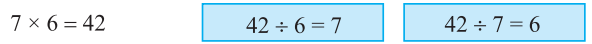Question 2.
9 × 4 = 36 __________ ____________
Solution:
36 ÷ 9 = 4, 36 ÷ 4 = 6Question 3.
6 × 8 = 48 __________ ____________
Solution:
48 ÷ 6 = 8, 48 ÷ 8 = 6

Question 4.
10 × 4 = 40 __________ ____________
Solution:
40 ÷ 10 = 4, 40 ÷ 4 = 10

2.

Question 1.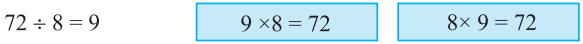Question 2.
35 ÷ 7 = 5 _____________ _____________
Solution:
5 × 7 = 35, 7 × 5 = 35

Question 3.
56 ÷ 8 = 7 _____________ _____________
Solution:
7 × 8 = 56, 8 × 7 = 56

Question 4.
150 ÷ 10 = 15 _____________ _____________
Solution:
10 × 15 = 150, 15 × 10 = 150Question 5.
120 ÷ 10 = 10 _____________ _____________
Solution:
10 × 12 = 120, 12 × 10 = 120

3. Divide and verify the solution :

Question 1.
66 ÷ 6
Solution: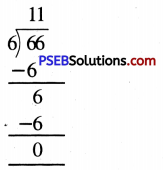Quotient = 11
Remainder = 0
Verification : Dividend = Quotient × Divisor + Remainder
66 = 11 × 6 + 0
66 = 66

Question 2.
431 ÷ 7
Solution: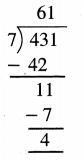Quotient = 61
Remainder = 4
Verification : Dividend = Quotient × Divisor + Remainder
431 = 61 × 7 + 4
431 = 427 + 4
431 = 431

Question 3.
728 ÷ 8
Solution: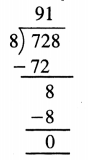Quotient = 91
Remainder = 0
Verification : Dividend = Quotient × Divisor + Remainder
728 = 91 × 8
728 = 728Question 4.
648 ÷ 9
Solution: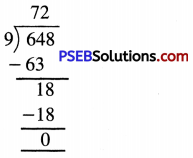Quotient = 72
Remainder = 0
Verification : Dividend = Quotient × Divisor + Remainder
648 = 72 × 9 + 0
648 = 648

Question 5.
960 ÷ 5
Solution: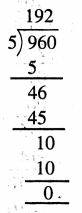Quotient = 192
Remainder = 0
Verification : Dividend = Quotient × Divisor + Remainder
960 = 192 × 5 + 0
960 = 960

4. Solve the following :

Question 1.
666 ÷ 6
Solution: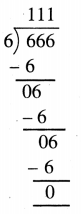Quotient = 111

Question 2.
655 ÷ 5
Solution: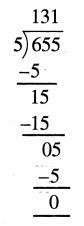Quotient = 131Question 3.
787 ÷ 7
Solution: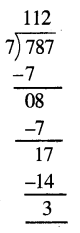Quotient = 112
Remainder = 3

Question 4.
877 ÷ 7
Solution: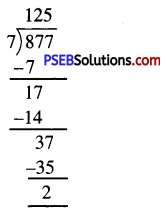Quotient = 125
Remainder = 2

Question 5.
598 ÷ 6
Solution: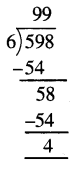Quotient = 99
Remainder = 4Question 6.
566 ÷ 8
Solution: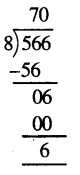Quotient = 70
Remainder = 6

Question 7.
707 ÷ 7
Solution: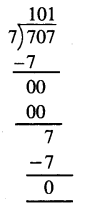Quotient = 101
Remainder = 0

5. Solve the following :

Question 1.
2150 ÷ 2
Solution: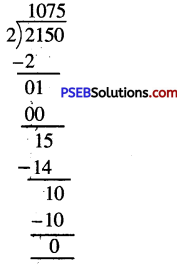Quotient = 1075

Question 2.
4050 ÷ 3
Solution: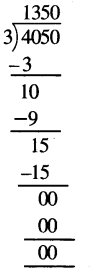Quotient = 1350Question 3.
8048 ÷ 8
Solution: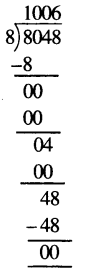Quotient = 1006

Question 4.
5106 ÷ 6
Solution: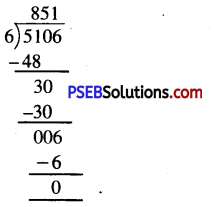Quotient = 851

Question 5.
3043 ÷ 3
Solution: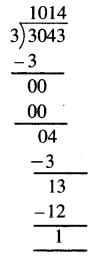Quotient = 1014
Remainder = 1

Question 6.
7890 ÷ 7
Solution: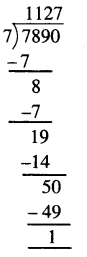Quotient = 1127
Remainder = 1Question 7.
4050 ÷ 5
Solution: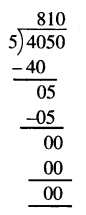Quotient = 810

6. Divide and verify the following:

Question 1.
96 ÷ 12
Solution: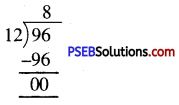Quotient = 8
Remainder = 0
Verification : Dividend = Quotient × Divisor + Remainder
96 = 8 × 12 + 0
96 = 96

Question 2.
98 ÷ 14
Solution: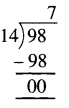Quotient = 7
Verification : Dividend = Quotient × Divisor + Remainder
98 = 14 × 7 + 0
98 = 98

Question 3.
78 ÷ 16
Solution: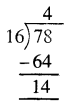Quotient = 4
Remainder = 14
Verification : Dividend = Quotient × Divisor + Remainder
78 = 4 × 16 + 14
78 = 64 + 14
78 = 78Question 4.
760 ÷ 19
Solution: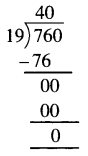Quotient = 40
Verification : Dividend = Quotient × Divisor + Remainder
760 = 40 × 19 + 0
760 = 760

Question 5.
550 ÷ 13
Solution: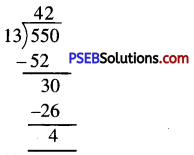Quotient = 42
Remainder = 4
Verification : Dividend = Quotient × Divisor + Remainder
550 = 42 × 13 + 4
550 = 446 + 4
550 = 550

Question 6.
894 ÷ 24
Solution: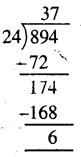Quotient = 37
Remainder = 6
Verification : Dividend = Quotient × Divisor + Remainder
894 = 37 × 24 + 6
894 = 888 + 6
894 = 894Question 7.
913 ÷ 66
Solution: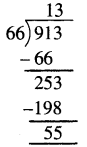Quotient = 13
Remainder = 55
Verification : Dividend = Quotient × Divisor + Remainder
913 = 13 × 66 + 55
913 = 858 + 55
913 = 913

Question 8.
826 ÷ 34
Solution: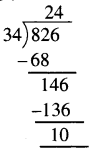Quotient = 24
Remainder = 10
Verification : Dividend = Quotient × Divisor + Remainder
826 = 24 × 34 + 10
826 = 816 + 10
826 = 826

Question 9.
7645 ÷ 12
Solution: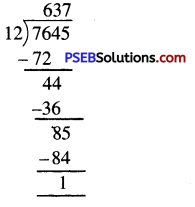Quotient = 637
Remainder = 1
Verification : Dividend = Quotient × Divisor + Remainder
7645 = 637 × 12 + 1
7645 = 7644 + 1
7645 = 7645

Question 10.
7813 ÷ 13
Solution: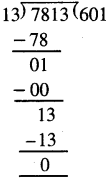Quotient = 601
Remainder = 0
Verification : Dividend = Quotient × Divisor + Remainder
7813 = 601 × 13 + 0
7813 = 7813Question 11.
5375 ÷ 25
Solution: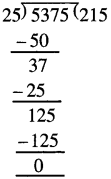Quotient = 215
Remainder = 0
Verification : Dividend = Quotient × Divisor + Remainder
5375 = 215 × 25 + 0
5375 = 5375

Question 12.
6767 ÷ 33
Solution: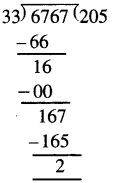Quotient = 205
Remainder = 2
Verification : Dividend = Quotient × Divisor + Remainder
6767 = 205 × 33 + 2
6767 = 6765 + 2
6767 = 6767Question 13.
9600 ÷ 50
Solution: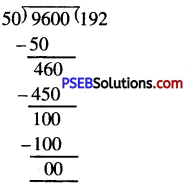Quotient = 192
Remainder = 0
Verification : Dividend = Quotient × Divisor + Remainder
9600 = 192 × 50 + 0
9600 = 9600

Question 14.
9999 ÷ 33
Solution: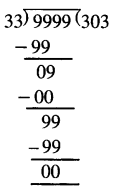Quotient = 303
Remainder = 0
Verification : Dividend = Quotient × Divisor + Remainder
9999 = 303 × 33 + 0
9999 = 9999Question 15.
9660 ÷ 60
Solution: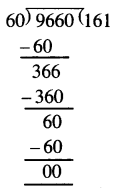Quotient = 161
Remainder = 0
Verification : Dividend = Quotient × Divisor + Remainder
9660 = 161 × 60 + 0
9660 = 9660